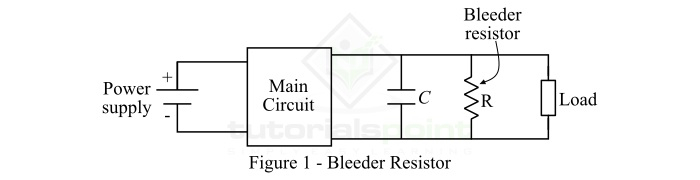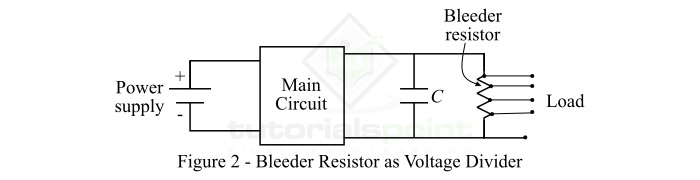# What is a Bleeder Resistor? – Definition, Formula, and Applications

## What is a Bleeder Resistor?

In electronic circuits, a resistor which is connected in parallel with the output of a high voltage power supply for discharging the filter capacitors of the power supply when the circuit is turned off is referred to as a bleeder resistor. It is also known as a leakage resistor or capacitor discharge resistor or safety discharge resistor.The bleeder resistor is primarily used for safety reasons because it eliminates the possibility of a left over charge that may cause an electric shock. Generally, a bleeder resistor is a standard resistor instead of a specialized resistor.

## Value of a Bleeder Resistor

The value of a bleeder resistor should be selected properly so that its presence in the circuit does not interfere the power supply and can discharge the filter capacitor in a short time.

However, if we choose a bleeder resistor of a small value, it will provide high speed bleeding, but it will consume more power. On the other hand, if we choose a bleeder resistor of a large value, then it will give less bleeding speed and less power consumption. Thus, the design engineer of the circuit has to choose a proper value of the bleeder resistor so that it is low enough to discharge the filter capacitor in a short time and high enough to not interfere with the power supply.

In order to determine the proper value of the bleeder resistor, consider the relationship between the discharging voltage and resistor as given below.

$$\mathrm{V=V_{m}\, e^{\frac{-t}{RC}}}$$

$$\mathrm{\Rightarrow \frac{V}{V_{m}}=\, e^{\frac{-t}{RC}}}$$

$$\mathrm{\Rightarrow ln\left ( \frac{V}{V_{m}} \right )=\frac{-t}{RC}}$$

$$\mathrm{\Rightarrow R=\frac{-t}{C\times ln\left ( \frac{V}{V_{m}} \right ) }}$$

Using this expression, we can determine the value of the bleeder resistor for safe voltage and time required to discharge the capacitor.

## Applications of Bleeder Resistor

The bleeder resistor is primarily used for safety purposes in electronic circuits. But, it can also be used in several other applications such as

• To improvement of the voltage regulation of a circuit.
• As a tapped resistor in voltage divider, etc.

Let us discuss the use of bleeder resistor for voltage regulation and voltage division.

### Bleeder Resistor for Voltage Regulation

The voltage regulation is given by the difference of no-load voltage and full-load voltage divided by the full-load voltage, i.e.

$$\mathrm{VR=\frac{V_{nl}-V_{fl}}{V_{fl}}}$$

Where, Vnl is the no-load voltage and Vfl is the full-load voltage.

For the better voltage regulation of the circuit, the value of the full-load voltage (Vfl) should be as low as possible.

When a bleeder resistor is connected in parallel with the filter capacitor. Then, a voltage drop will occur in the bleeder resistor along with the load resistor. When the power supply is ON, a voltage drop occurs in the load resistor, and when the power supply is OFF, a voltage drop occurs in the bleeder resistor. In this way, the bleeder resistor increases the value of the noload voltage. Consequently, the difference of the no-load voltage and the full-load voltage is reduced that improves the voltage regulation of the circuit.

### Bleeder Resistor for Voltage Division

The bleeder resistor is also used as a tapped resistor to provide multiple voltages from a single voltage. The circuit configuration of the bleeder resistor as a voltage divider is shown in the following figure.In the given circuit, the bleeder resistor has four tapping points. By using a proper tap point, we can obtain four different voltages across the load. In this way, the bleeder resistor may also serve as a voltage divider.

## Conclusion

From the above discussion, we may conclude that the bleeder resistor is a standard resistor that is used parallel with the filter capacitor of a power supply to discharge the filter capacitor when the circuit is turned off. We may use a bleeder resistor to improve the voltage regulation of a circuit and to divide a single voltage into multiple values.